[This article was first published on r-bloggers – WZB Data Science Blog, and kindly contributed to R-bloggers]. (You can report issue about the content on this page here)
Want to share your content on R-bloggers? click here if you have a blog, or here if you don't.

In many scenarios, data are structured in groups or clusters, e.g. pupils within classes (within schools), survey respondents within countries or, for longitudinal surveys, survey answers per subject. Simply ignoring this structure will likely lead to spuriously low standard errors, i.e. a misleadingly precise estimate of our coefficients. This in turn leads to overly-narrow confidence intervals, overly-low p-values and possibly wrong conclusions.

Clustered standard errors are a common way to deal with this problem. Unlike Stata, R doesn’t have built-in functionality to estimate clustered standard errors. There are several packages though that add this functionality and this article will introduce three of them, explaining how they can be used and what their advantages and disadvantages are. Before that, I will outline the theory behind (clustered) standard errors for linear regression. The last section is used for a performance comparison between the three presented packages. If you’re already familiar with the concept of clustered standard errors, you may skip to the hands-on part right away.

## Data

We’ll work with the dataset nlswork that’s included in Stata, so we can easily compare the results with Stata. The data comes from the US National Longitudinal Survey (NLS) and contains information about more than 4,000 young working women. As for this example, we’re interested in the relationship between wage (here as log-scaled GNP-adjusted wage) as dependent variable (DV) ln_wage and survey participant’s current age, job tenure in years and union membership as independent variables. It’s a longitudinal survey, so subjects were asked repeatedly between 1968 and 1988 and each subject is identified by an unique idcode.

The example data is used for illustrative purposes only and we skip many things that we’d normally do, such as investigating descriptive statistics and exploratory plots. To keep the data size limited, we’ll only work with a subset of the data (only subjects with IDs 1 to 100) and we also simply dismiss any observations that contain missing values.

library(webuse)
library(dplyr)

nlswork_orig <- webuse('nlswork')

nlswork <- filter(nlswork_orig, idcode <= 100) %>%
select(idcode, year, ln_wage, age, tenure, union) %>%
filter(complete.cases(.)) %>%
mutate(union = as.integer(union),
idcode = as.factor(idcode))
str(nlswork)
tibble [386 × 6] (S3: tbl_df/tbl/data.frame)
$idcode : Factor w/ 82 levels "1","2","3","4",..: 1 1 1 1 1 1 1 2 2 2 …$ year   : num [1:386] 72 77 80 83 85 87 88 71 77 78 …
$ln_wage: num [1:386] 1.59 1.78 2.55 2.42 2.61 …$ age    : num [1:386] 20 25 28 31 33 35 37 19 25 26 …
$tenure : num [1:386] 0.917 1.5 1.833 0.667 1.917 …$ union  : int [1:386] 1 0 1 1 1 1 1 0 1 1 …

Let’s have a look at the first few observations. They contain data from subject #1, who was surveyed several times between 1972 and 1988, and a few observations from subject #2.

idcode  year ln_wage   age tenure union
1         72    1.59    20  0.917     1
1         77    1.78    25  1.5       0
1         80    2.55    28  1.83      1
1         83    2.42    31  0.667     1
1         85    2.61    33  1.92      1
1         87    2.54    35  3.92      1
1         88    2.46    37  5.33      1
2         71    1.36    19  0.25      0
2         77    1.73    25  2.67      1
2         78    1.69    26  3.67      1

A summary of all but the idcode variable gives the following:

   year          ln_wage            age           tenure           union
Min.   :70.00   Min.   :0.4733   Min.   :18.0   Min.   : 0.000   Min.   :0.0000
1st Qu.:73.00   1st Qu.:1.6131   1st Qu.:25.0   1st Qu.: 1.167   1st Qu.:0.0000
Median :80.00   Median :1.9559   Median :31.0   Median : 2.417   Median :0.0000
Mean   :79.61   Mean   :1.9453   Mean   :30.8   Mean   : 3.636   Mean   :0.2591
3rd Qu.:85.00   3rd Qu.:2.2349   3rd Qu.:36.0   3rd Qu.: 4.958   3rd Qu.:1.0000
Max.   :88.00   Max.   :3.5791   Max.   :45.0   Max.   :19.000   Max.   :1.0000 

We have 82 subjects in our subset and the number of times each subject was surveyed ranges from only once to twelve times:

summary(as.integer(table(nlswork$idcode))) Min. 1st Qu. Median Mean 3rd Qu. Max. 1.000 2.000 4.000 4.707 7.000 12.000  In more than one quarter of the observations, the subject answered to be currently member of a trade union. The following shows the distribution of the DV in our data. It is roughly normally distributed with mean 1.95 and standard deviation (SD) 0.45. We can calculate the mean and SD of the DV separately for each subject. A histogram of these subject-specific means reveals more variability: y_mean_sd_cl <- sapply(levels(nlswork$idcode), function(idcode) {
y_cl <- nlswork$ln_wage[nlswork$idcode == idcode]
c(mean(y_cl), sd(y_cl))
})
hist(y_mean_sd_cl[1,], breaks = 20,
main = 'Histogram of DV means per subject', xlab = NA)

We can compare the SD of the subject-specific means with the mean of the SDs calculated from each subjects’ repeated measures.

c(sd(y_mean_sd_cl[1,]), mean(y_mean_sd_cl[2,], na.rm = TRUE))
 0.4038449 0.2221142

The SD between the subject-specific means is almost twice as large as the mean of the SD from each subjects’ values. This shows that there’s much more variability between each subject than within each subject’s repeated measures regarding the DV.

## Fixed-effects model, not adjusting for clustered observations

Our data contains repeated measures for each subject, so we have panel data in which each subject forms a group or cluster. We can use a fixed-effects (FE) model to account for unobserved subject-specific characteristics. We do so by including the subject’s idcode in our model formula. It’s important to note that idcode is of type factor (we applied idcode = as.factor(idcode) when we prepared the data) so that for each factor level (i.e. each subject) an FE coefficient will be estimated that represents the subject-specific mean of our DV. 1

Let’s specify and fit such a model using lm. We include job tenure, union membership and an interaction between both (the latter mainly for illustrative purposes later when we estimate marginal effects). We also control for age and add idcode as FE variable.

m1 <- lm(ln_wage ~ age + tenure + union + tenure:union + idcode,
data = nlswork)
summary(m1)
Call:
lm(formula = ln_wage ~ age + tenure + union + tenure:union +
idcode, data = nlswork)
Residuals:
Min       1Q   Median       3Q      Max
-0.96463 -0.09405  0.00000  0.11460  1.23525
Coefficients:
Estimate Std. Error t value Pr(>|t|)
(Intercept)   1.882e+00  1.314e-01  14.325  < 2e-16 ***
age           5.631e-03  3.110e-03   1.811 0.071193 .
tenure        2.076e-02  6.964e-03   2.980 0.003115 **
union         1.746e-01  6.065e-02   2.879 0.004272 **
idcode2      -6.174e-01  1.285e-01  -4.803 2.47e-06 ***
idcode3      -5.625e-01  1.329e-01  -4.234 3.05e-05 ***
[...]

We’re not really interested in the subject-specific means (the FE coefficients), so let’s filter them out and only show our coefficients of interest:

m1coeffs_std <- data.frame(summary(m1)$coefficients) coi_indices <- which(!startsWith(row.names(m1coeffs_std), 'idcode')) m1coeffs_std[coi_indices,] Estimate Std..Error t.value Pr...t.. (Intercept) 1.882478232 0.131411504 14.325064 8.022367e-36 age 0.005630809 0.003109803 1.810664 7.119315e-02 tenure 0.020756426 0.006964417 2.980353 3.114742e-03 union 0.174619394 0.060646038 2.879321 4.272027e-03 tenure:union 0.014974113 0.009548509 1.568215 1.178851e-01 Unsurprisingly, job tenure and especially union membership are positively associated with wage. The coefficient of the interaction term shows that with union membership the job tenure effect is even a bit higher, though not significantly. In the next two sections we’ll see how standard errors for our estimates are usually computed and how this fits into a framework called “sandwich estimators.” Using this framework, we’ll see how the standard error calculations can be adjusted for clustered data. ## Standard errors In ordinary least squares (OLS) regression, we assume that the regression model errors are independent. This is not the case here: Each subject may be surveyed several times so within each subject’s repeated measures, the errors will be correlated. Although that is not a problem for our regression estimates (they are still unbiased [Roberts 2013]), it is a problem for for the precision of our estimates — the precision will typically be overestimated, i.e. the standard errors (SEs) will be lower than they should be [Cameron and Miller 2013]. The intuition behind this regarding our example is that within our clusters we usually have lower variance since the answers come from the same subject and are correlated. This lowers our estimates’ SEs. We can deal with this using clustered standard errors with subjects representing our clusters. But before we do this, let’s first have a closer look on how “classic” OLS estimates’ SEs are actually computed. In matrix notation, a linear model has the formThis model hasparameters (including the intercept parameter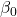) expressed asparameter vector. The parameters will be estimated fromobservations in our data. The DV is(anvector), the independent variables form an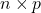matrix. Finally, the error termis anvector that captures everything that influencesbut cannot be explained by. In classic OLS, we assume thathas a mean of zero and a variance of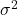. By minimizingan estimation for our parameters,, can be found as. The estimated variancefor these parameters is then (1)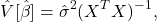whereis the estimated variance of the error or residual variance. This is calculated as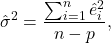withbeing the residuals. The numerator is also called the residual sum of squares and the denominator is the degrees of freedom. You can see for example in Sheather 2009 for how the formulas forandare derived or watch this video for a clear step-by-step derivation. We now have all the pieces together to replicate the standard errors from model m1 with our own calculations. To translate these formulae to R, we use model.matrix to get the design matrix, residuals for the residual vector, nobs for the number of observations, ncol(X) for the number or parameters, solve to calculate the inverse ofand diag to extract the diagonal of a square matrix. X <- model.matrix(m1) u <- residuals(m1) n <- nobs(m1) p <- ncol(X) sigma2 <- sum(u^2) / (n - p) # solve (X^T X) A = I, where I is identity matrix -> A is (X^T X)^-1 crossXinv <- solve(t(X) %*% X, diag(p)) m1se <- sqrt(diag(sigma2 * crossXinv)) m1se  0.131411504 0.003109803 0.006964417 0.060646038 0.128532897 ... Let’s check if this is equal to the standard errors calculated by lm (using near because of minor deviations due to floating point number imprecision): all(near(m1se, m1coeffs_std$Std..Error))
 TRUE

We extracted our parameter estimates’ variancefrom the diagonal of the (variance-)covariance or vcov matrix and R has the vcov function to calculate it from a fitted model. It’s exactly what we computed before using eq. 1:

all(near(sigma2 * crossXinv, vcov(m1)))
 TRUE

## Clustered standard errors

Classic OLS SEs can be generalized so that some assumptions, namely that the regression model errors are independent, can be relaxed. The foundation for this is the sandwich estimator 2

(2)Let’s first understand how the above equation relates to eq. 1, the classic OLS parameter variance: One assumption of classic OLS is constant variance (or homoscedasticity) in the errors across the full spectrum of our DV. This implicates that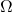is a diagonal matrix with identicalelements, i.e.. Plugging this into eq. 2 gives eq. 1 which shows that the classic OLS parameter variance is a specialized form of the sandwich estimator. 3

When we want to obtain clustered SEs, we need to consider thatin the “meat” part of eq. 2 is not a diagonal matrix with identicalelements anymore, hence this can’t be simplified to eq. 1. Instead, we can assume thatis block-diagonal with the clusters forming the blocks. This means we assume that the variance in the errors is constant only within clusters and so we first calculateper cluster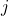and then sum the. Cameron and Miller 2013 (p. 11) show howis calculated in detail and also which finite-sample correction factor is applied. From this article we get the equation

(3)where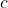is the number of clusters. It’s interesting to see how the residuals are added up per cluster and then averaged. As Cameron and Miller 2013 (p. 13) notes, this implicates an important limitation: With a low number of clusters, this averaging is imprecise.

Let’s translate this formula to R. We already haveas u (the residuals) and the design matrix X. We can generate a list of matrices, sum them and multiply the correction factor:

omegaj <- lapply(levels(nlswork$idcode), function(idcode) { j <- nlswork$idcode == idcode
# drop = FALSE: don't drop dimensions when we have only one obs.
X_j <- X[j, , drop = FALSE]
# tcrossprod is outer product x * x^T
t(X_j) %*% tcrossprod(u[j]) %*% X_j
})

## Option 3: lm_robust from estimatr

Another option is to use lm_robust from the estimatr package which is part of the DeclareDesign framework [Blair et al. 2019]. Like lm.cluster, it’s more convenient to use, but it doesn’t rely on sandwich and lmtest in the background and instead comes with an own implementation for model fitting and covariance estimation. This implementation is supposed to be faster than the other approaches and we’ll check that for our example later.

But first, let’s fit a model with clustered SEs using lm_robust. We use the same formula as with lm or lm.cluster, but also specify the clusters parameter: 5

library(estimatr)

m3 <- lm_robust(ln_wage ~ age + tenure + union + tenure:union + idcode,
clusters = idcode,
data = nlswork)
summary(m3)
Call:
lm_robust(formula = ln_wage ~ age + tenure + union + tenure:union +
idcode, data = nlswork, clusters = idcode)
Standard error type:  CR2
Coefficients:
Estimate Std. Error    t value  Pr(>|t|)   CI Lower  CI Upper     DF
(Intercept)   1.882e+00   0.141424  13.310872 8.596e-14  1.5930761  2.171880 28.642
age           5.631e-03   0.005726   0.983442 3.342e-01 -0.0061215  0.017383 26.790
tenure        2.076e-02   0.010089   2.057345 5.771e-02 -0.0007714  0.042284 14.812
union         1.746e-01   0.093790   1.861817 7.735e-02 -0.0209918  0.370231 20.049
idcode2      -6.174e-01   0.019094 -32.334722 2.810e-07 -0.6656871 -0.569086  5.283
idcode3      -5.625e-01   0.078607  -7.156034 9.687e-07 -0.7273116 -0.397718 18.550

Unlike lm, lm_robust allows to specify fixed effects in a separate fixed_effects formula parameter which, according to the documentation, should speed up computation for many types of SEs. Furthermore, this cleans up the summary output since there are no more FE coefficients:

m3fe <- lm_robust(ln_wage ~ age + tenure + union + tenure:union,
clusters = idcode,
fixed_effects = ~idcode,
data = nlswork)
summary(m3fe)
Call:
lm_robust(formula = ln_wage ~ age + tenure + union + tenure:union,
data = nlswork, clusters = idcode, fixed_effects = ~idcode)
Standard error type:  CR2
Coefficients:
Estimate Std. Error t value Pr(>|t|)   CI Lower CI Upper     DF
age          0.005631   0.005726  0.9834  0.33419 -0.0061215  0.01738 26.790
tenure       0.020756   0.010089  2.0573  0.05771 -0.0007714  0.04228 14.812
union        0.174619   0.093790  1.8618  0.07735 -0.0209918  0.37023 20.049
tenure:union 0.014974   0.009043  1.6558  0.14062 -0.0062982  0.03625  7.187
Multiple R-squared:  0.7554 ,    Adjusted R-squared:  0.6861
[...]

When we compare the results from lm_robust with lm, we can see that the point estimates are the same. The lm_robust SEs are, as expected, higher than the “classic” SEs from lm. However, the lm_robust SEs are also a bit smaller than those calculated from sandwich::vcovCL:

m3fe_df <- tidy(m3fe) %>% rename(est.lm_robust = estimate,
se.lm_robust = std.error)
m3fe_df$se.sandwich <- m1coeffs_cl[coi_indices,2][2:5] m3fe_df$est.classic <-  m1coeffs_std[coi_indices,1][2:5]
m3fe_df$se.classic <- m1coeffs_std[coi_indices,2][2:5] m3fe_df[c('term', 'est.classic', 'est.lm_robust', 'se.classic', 'se.lm_robust', 'se.sandwich')] term est.classic est.lm_robust se.classic se.lm_robust se.sandwich age 0.005630809 0.005630809 0.003109803 0.005725617 0.006339777 tenure 0.020756426 0.020756426 0.006964417 0.010088940 0.011149190 union 0.174619394 0.174619394 0.060646038 0.093789776 0.101970509 tenure:union 0.014974113 0.014974113 0.009548509 0.009043429 0.009646023 This is because lm_robust by default uses a different cluster-robust variance estimator “to correct hypotheses tests for small samples and work with commonly specified fixed effects and weights” as explained in the Getting started vignette. Details can be found in the Mathematical notes for estimatr. As with the lm and lm.cluster results, we can also estimate marginal effects with a lm_robust result object. However, this doesn’t seem to work when you specify FEs via fixed_effects parameter as done for m3fe: margins(m3fe, variables = 'tenure', at = list(union = 0:1)) %>% summary() Error in predict.lm_robust(model, newdata = data, type = type, se.fit = TRUE, : Can't set se.fit == TRUE with fixed_effects With m3 (where FEs were directly specified in the model formula), marginal effects estimation works and we don’t even need to pass a separate vcov matrix, since this information already comes with the lm_robust result object m3. 6 margins(m3, variables = 'tenure', at = list(union = 0:1)) %>% summary() factor union AME SE z p lower upper tenure 0.0000 0.0208 0.0101 2.0573 0.0397 0.0010 0.0405 tenure 1.0000 0.0357 0.0077 4.6174 0.0000 0.0206 0.0509 As already said, lm_robust uses a different variance estimator than sandwich’s vcovCL and Stata. However, by setting se_type to 'stata' we can replicate these “Stata Clustered SEs”: m3stata <- lm_robust(ln_wage ~ age + tenure + union + tenure:union + idcode, clusters = idcode, se_type = 'stata', data = nlswork) m3stata_se <- tidy(m3stata) %>% pull(std.error) all(near(m3stata_se, m1clse)) # same SEs?  TRUE In summary, lm_robust is as convenient to use as lm or lm.cluster, but offers similar flexibility as sandwich for estimating clustered SEs. A big advantage is that you don’t need to care about supplying the right covariance matrix to further post-estimation functions like margins. The proper covariance matrix is directy attached to the fitted lm_robust object (and can be accessed via model$vcov or vcov(model) if you need to). Is parameter estimation also faster with lm_robust?

## Performance comparison

We’ll make a rather superficial performance comparison only using the nlswork dataset and microbenchmark. We will compare the following implementations for estimating model coefficients and clustered SEs:

1. lm and vcovCL from sandwich
2. lm.cluster
3. lm_robust with default SEs (se_type = 'CR2')
4. lm_robust with Stata SEs (se_type = 'stata')
5. lm_robust with fixed_effects parameter and Stata SEs (fixed_effects = idcode, se_type = 'stata')

For a fair comparison, we don’t calculate CIs (which lm_robust by default does). These are the results for 100 test runs:

As expected, lm/sandwich and lm.cluster have similar run times. lm_robust is faster for all three configurations (3. to 5.) and is especially fast when estimating Stata SEs (4. and 5.). With our example data, specifying fixed_effects (5.) doesn’t seem to speed up the calculations.

## Conclusion

We’ve seen that it’s important to account for clusters in data when estimating model parameters, since ignoring this fact will likely result in overestimated precision which in turn can lead to wrong inference. R provides many ways to estimate clustered SEs. The packages sandwich and lmtest come with a rich set of tools for this task (and also for other types of robust SEs) and work with lm and other kinds of models. lm.cluster from the miceadds package provides a more convenient wrapper around sandwich and lmtest. However, users should be careful to not forget passing along the separate cluster robust covariance matrix for post-estimation tasks. This is something users don’t need to care for when using lm_robust from the estimatr package, since the covariance matrix is not separate from the fitted model object. Another advantage is that lm_robust seems to be faster than the other options.

## References

Blair, Graeme, Jasper Cooper, Alexander Coppock, and Macartan Humphreys. 2019. “Declaring and Diagnosing Research Designs.” American Political Science Review 113 (3): 838–59.

Cameron, A Colin, and Douglas L Miller. 2013. “A Practitioner’s Guide to Cluster-Robust Inference.”

Roberts, Molly. 2013. “Robust and Clustered Standard Errors.”

Sheather, Simon. 2009. A Modern Approach to Regression with R. Springer Texts in Statistics. New York: Springer-Verlag.

Zeileis, Achim, Susanne Köll, and Nathaniel Graham. 2020. “Various Versatile Variances: An Object-Oriented Implementation of Clustered Covariances in R.” Journal of Statistical Software 95 (1).

1.

It is not always necessary to use an FE model and you can very well estimate robust SEs from clustered data also without FEs.

2.

This is called sandwich estimator because of the structure of the formula: Between two slices of bread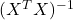there is the meat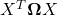3.

Plugging inin eq. 2 giveswhich reduces to eq. 1. because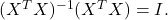4.

Once again a strange package name. Unlike sandwich this package name is not derived from a formula, but simply stands for additional functionality for imputation with the mice package — and just happens to include clustered SE estimation.

5.

Note that this time we have to use the “bare” unquoted variable name — not a formula, not a string. Every package has a different policy!

6.

The standard vcov function will return the correct (cluster robust) covariance matrix for a fitted lm_robust model, whereas it will return the “classic” covariance matrix for a fitted lm model.